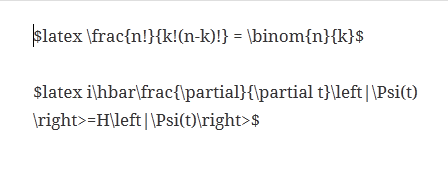## Beautiful Maths$\frac{n!}{k!(n-k)!} = \binom{n}{k}$$i\hbar\frac{\partial}{\partial t}\left|\Psi(t)\right>=H\left|\Psi(t)\right>$